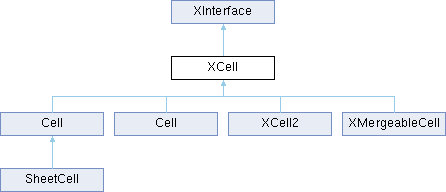LibreOffice LibreOffice 7.4 SDK API Reference
XCell Interface Referencepublished

provides methods to access the contents of a cell in a table. More...

`import"XCell.idl";`

Inheritance diagram for XCell:## Public Member Functions

string getFormula ()
returns the formula string of a cell. More...

void setFormula ([in] string aFormula)
sets a formula into the cell. More...

double getValue ()
returns the floating point value of the cell. More...

void setValue ([in] double nValue)
sets a floating point value into the cell. More...

com::sun::star::table::CellContentType getType ()
returns the type of the cell. More...

long getError ()
returns the error value of the cell. More...Public Member Functions inherited from XInterface
any queryInterface ([in] type aType)
queries for a new interface to an existing UNO object. More...

void acquire ()
increases the reference counter by one. More...

void release ()
decreases the reference counter by one. More...

## Detailed Description

provides methods to access the contents of a cell in a table.

com::sun::star::table::Cell

## ◆ getError()

 long getError ( )

returns the error value of the cell.

If the cell does not contain a formula, the error is always zero.

## ◆ getFormula()

 string getFormula ( )

returns the formula string of a cell.

Even if the cell does not contain a formula, an assignment of this attribute's value to another cell's formula attribute would create the same cell content. This is because this attribute contains the original text value of a string cell. The value of a value cell will be formatted using the number format's default format or the formula string, including "=", of a formula cell.

## ◆ getType()

 com::sun::star::table::CellContentType getType ( )

returns the type of the cell.

## ◆ getValue()

 double getValue ( )

returns the floating point value of the cell.

For a value cell the value is returned, for a string cell zero is returned and for a formula cell the result value of a formula is returned.

## ◆ setFormula()

 void setFormula ( [in] string aFormula )

sets a formula into the cell.

When assigned, the string will be interpreted and a value, text or formula cell is created, depending on the text and the number format.

## ◆ setValue()

 void setValue ( [in] double nValue )

sets a floating point value into the cell.

After a call to this method the type of the cell is CellContentType::VALUE.

The documentation for this interface was generated from the following file: